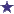# Days Supply Question - 480 ml solution: 15 ml by mouth every 6 hours as needed480 ML Liquid Medication Solution - What is days supply with these directions?

What is the days supply in this question?

### Comments for Days Supply Question - 480 ml solution: 15 ml by mouth every 6 hours as needed

Average RatingSep 16, 2017 Rating8 Days Supply by: David "480 ml solution: 15 ml by mouth every 6 hours as needed" First, we figure out the maximum amount of volume that the patient can use in one day, and then, second, we would then divide that volume into 480 milliliters, which is the total amount of the prescription. 15 ml X 4 (4 times daily, or every 6 hours) = 60 ml max total volume daily. 480 ml divided by 60 ml = 8 days supply